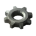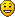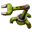## Factorio and real physics

Post all other topics which do not belong to any other category.
ssilk
Global ModeratorPosts: 12817
Joined: Tue Apr 16, 2013 10:35 pm
Contact:

### Factorio and real physics

When I worked on the test track I found out, that many physics in the game is some like compareable to reality.

Work

The definition of work is force multiplied with distance. So in factorio! We need to replace the force with items. This is explainable, items have a weight, they don't move themselves, to move them a force is needed. (It can be explained also differently: in quantum physics things doesn't exist any more, instead there are only some strange forces left. The same is with items: when I look into the data structures only some bit and byte and functions are left. Ok - it's just a game!)
And the distance is logically measured in tiles.
Now we have items * tiles. Item tiles.
Moving an item makes only sense, if you tell, how fast, or how long it takes to reach, so you need to multiply with time.
This is 1 work: move an item a tile per second.
In physics you have this equivalence: 1 newton meter = 1 joule
In factorio it is: 1 item tile = 1 work

Speed

Tiles per Second. Belts for example move with 1.8 tiles/sec

Energy

1 newton meter is also 1 watt second
Factorio has its own rules, of how much energy is used to do this and that, but it might be a rule of thumb for new stuff, new items etc. to estimate the use of energy when you compare 1 item tile = 1 work = 1 watt second.

Example: 100 bots can take 2 item each and drive 3 tiles per second, so the work is 100*2*3 = 600 item tiles per second 36000 wattseconds /minute. Which is 2160000 watt per hour or 2160kWH!
(So logistic robots might need a significant amount of energy?!

We can calculate that also for belts, inserters and I would also assemblies: they are 3x3 tiles, so the work is 9 times bigger! So when an assembly needs 2 steel plate and outputs a wheel in a second we have 3 item x 9 tiles = 27 item tiles. Which is of course too less, but we also said already , that the assemblies are too small.and again: it might help to estimate the right amount of energy, nothing more!

Flow/throughput

The flow is how many items per time. A basic belt has for example a maximum flow of 720 items per minute.

Capacity

A chest contains stacks and a stack has different sizes and can contain a different number of items depending on their size.
So, we have here two variables: stack size and item size. What capacity wants to say is how much items of this type are going in? and so, the capacity is measured in items per item type.

Do you have more ideas?

Conclusion

We can calculates some useful numbers for logistic bots, belts and trains out of that!

- the "work" the logistics can make as theoretical maximum. Items per tiles in a minute or how many items can be transported one tile in a minute.
See the example above. The 100 logistic bots can transport 36000 item tiles per minute.
- the same can be calculated as real statistics, the real transported items tiles. For example a bot has transported 2 items 50 tiles wide, then he returns empty. The resulting work is 2*50 + 0*50 = 100 item tiles.
- the percentage between theory and practice. At a later version some warnings can lit up, when a minimum is reached.
Example: in theory the bot can transport 2 items 100 tiles wide. Practical he manages only 50. So 50/100 = 0.5 or 50%
I think/estimate under a limit of 60% the logistic bots begin to be useless.
Cool suggestion: Eatable MOUSE-pointers.
Have you used the Advanced Search today?
Need help, question? FAQ - Wiki - Forum help
I still like small signatures...

n9103
Smart InserterPosts: 1067
Joined: Wed Feb 20, 2013 12:09 am
Contact:

### Re: Factorio and real physics

item tiles = "aisles/isles"? :p

An unusual take on the math, though there were several errors you made. Those I particularly noticed:
You make a jump from comparing work units to aisles when you got to talking about bots. (The resulting work is 2*50 + 0*50 = 100 item tiles.) And as you pointed out, item tiles themselves are meaningless without a timeframe.
You work with comparisons of a single belt vs 100 bots.
You didn't specify if the bots were or weren't upgraded. (assumption would be not, since the belt was a basic.)
As Bots are rarely used without upgrades, and factories rarely use only basic belts by the time bots are in good supply, comparing basic to basic seems irrelevant.
Bots don't travel in purely Cardinal directions, whereas belts do. A mid-point between maximum and minimum inefficiency for belts' cardinal limitation should be used in a comparison to bots.

Otherwise, another interesting entry in your series on the math behind Factorio.
Colonel Failure wrote:You can lose your Ecologist Badge quite quickly once you get to the point of just being able to murder them willy-nilly without a second care in the world.

ssilk
Global ModeratorPosts: 12817
Joined: Tue Apr 16, 2013 10:35 pm
Contact:

### Re: Factorio and real physics

Edit: I replaced "power" with "t'power" and "work" with "t'work", to avoid conflicts with electrical power...

Edit-2: Rewrote some misunderstandable parts.
n9103 wrote:item tiles = "aisles/isles"? :p
I think this is a proverb? In German we say "you replaced apples with trees" or "what is it? Bratwursts?"...

item*tiles = itemtiles, you can remove the multiplication sign. On iPad the spell correction automatically replaces it as "item tile".An unusual take on the math, though there were several errors you made. Those I particularly noticed:
You make a jump from comparing work units to aisles when you got to talking about bots. (The resulting work is 2*50 + 0*50 = 100 item tiles.) And as you pointed out, item tiles themselves are meaningless without a timeframe.
Well, it took me a while to come to my fail: I mixed up work and power. I totally forgot that - it was late and I couldn't sleep.

So, lets repeat the calculations:
2 items * 50 tiles + 0 items * 50 tiles = 100 itemtiles
100 itemtiles is the transport-power!

It's like Watt (=power) vs. Wattseconds (=work). E. g. my toaster needs 2000 Watt. This means, that he uses 2000 Wattseconds, if he runs one second. Or 2 kWh for one hour.

So when I have for example bots which can transport 2 items each and fly 3 tiles per second, they have a theoretical maximum transportation-POWER. But when I look, how many tiles did they transport within a given time, then it is t'work. When I look, how many items are currently transported, in this moment, then it is t'power, when I look for that over a longer time, it is t'work.

100 [bots] * 2 items 3 tiles/sec = 100 items*tiles/sec

And as long as this "/sec" is there, we can call it "t'power". That's like the physical definition of power. But when I need the t'work I need to multiply it with seconds:

100 ( items * tiles / sec ) * 10 secs = 1000 itemtiles
You work with comparisons of a single belt vs 100 bots.
You didn't specify if the bots were or weren't upgraded. (assumption would be not, since the belt was a basic.)
Comparison? Eh? Don't know what you mean... but comparing belts with bots is a good idea!
A basic belt can transport 720 items/minute or 12 per second. This is not dependend from the distance, or the distance (tile) is always 1. So we can say a belt has a transportation-power of 12, which is also the t'work it does in a second. So a belt of 10 tiles has the t'power of 120. and so on.

So lets calculate, when logistic bots are better than belts. The problem is here, that I currently don't know how fast a logistic bot really is. I estimate 3 tiles per second, but per sure it's slower/faster. But if known the real numbers can be replaced. And I calculate, that the bots take 2 items each. How many bots are needed, to have the same transportaton-power than a belt over 10 tiles?

120 itemtiles/sec = 2 items * 3 tiles/sec * bots
=> I divide thru bots and with 120 itemtiles/sec:
1/bots = (2 items * 3 tiles/sec) / (120 itemtiles/sec) = 6 itemtiles/sec / 120 itemtiles/sec
=> we can cut the units and invers:
bots = 120/6 = 20
=> ...you need 20 bots for that. And we can obtain a formula to replace a belt with bots:

needed bots = t'power of the belt / t'power of a bot
where
t'power of a bot = itemloadcapacity * speed (both of the bot of course)
t'power of belt = length * flow

This assumes of course, that the track is the same. But don't can have all. I find this very interesting.Edit:
To correct the last points of my first post here:

- We can calculate the theoretical transportation-power of the logistic bots (this is not t'work as written in the first post).
. Lets assume, we can look, how many bots are currently transporting (and how many items), we have also the real transportation-power of the logistic bots.
- Lets assume, we do that every second and make nice graphs with that: That what is under the line in sum is the t'work they did!
- The t'work-average of the bots is for example, if we take the last 10 secs, the last 60 secs etc. of that. That unit is also t'work.
- The division between the t'work in a given time-interval and the maximal t'power in the same interval is the load-average of the logistic-system.

I would like to see that numbers like in the electric network-info.

I would like to have measure-points (how many items are going thru here since start, what flow is it per second? Connect it with a second point and because they know their distance, the t'work, t'power etc. can be calculated.
Or an area. I select an area on the map and it calculates the belts on it and their type and calculate the theoretical max. transport-power of that.
As Bots are rarely used without upgrades, and factories rarely use only basic belts by the time bots are in good supply, comparing basic to basic seems irrelevant.
Hm. It was just an example. And I proved, that two basic belts have more t'power than a fast. want to give the readers the knowledge, how to calculate it themselves on the real number.
Bots don't travel in purely Cardinal directions, whereas belts do. A mid-point between maximum and minimum inefficiency for belts' cardinal limitation should be used in a comparison to bots.
It would be a nice excel-table: When are belts more efficient than bots? When is train more useful?Hard work to do that all.
Otherwise, another interesting entry in your series on the math behind Factorio.
Thanks.
I must repeat here the reason why I do this: Factorio has much to do with engineering. Or "form follows function". The most useful layout of something should be calculateable or proveable if it is really slower or faster than another layout. Currently we can only built it, but how would it be, if I just can say: I need to transport X from Y to Z. And I get the answer: Use belts, Luke! And to top this, the belt is created in the most efficient way. (dreaming)
Cool suggestion: Eatable MOUSE-pointers.
Have you used the Advanced Search today?
Need help, question? FAQ - Wiki - Forum help
I still like small signatures...

FreeER
Smart InserterPosts: 1266
Joined: Mon Feb 18, 2013 4:26 am
Contact:

### Re: Factorio and real physics

In Factorio (0.7.x at least) The speed of the logistic robot speed is equal to the speed in the prototype (0.05) * (SpeedModifier+1) per tick, so 3 per second with no upgrades. So t'power of a vanilla bot = (1+CargoModifier)(0.05*(1+SpeedModifier)
The speed of a Transport belt 'seems' to be exactly what is specified in the prototype (thus 1.875 per second for a basic belt, 3.75 for fast, and 5.625 for express) at least when traveling straight (no turns).

edit: looking through the wiki page https://forums.factorio.com/wiki/inde ... sport_belt (which is where I can from, I had clicked the link from belt physics without reading the rest and then started looking at code lol) it seems like this has been determined alreadyoh well, apologize for the bump I guess
<I'm really not active any more so these may not be up to date>
~FreeER=Factorio Modding
- Factorio Wiki
- My Factorio Modding Guide
- Wiki Modding Guide
Feel free to pm meOr drop into #factorio on irc.esper.net